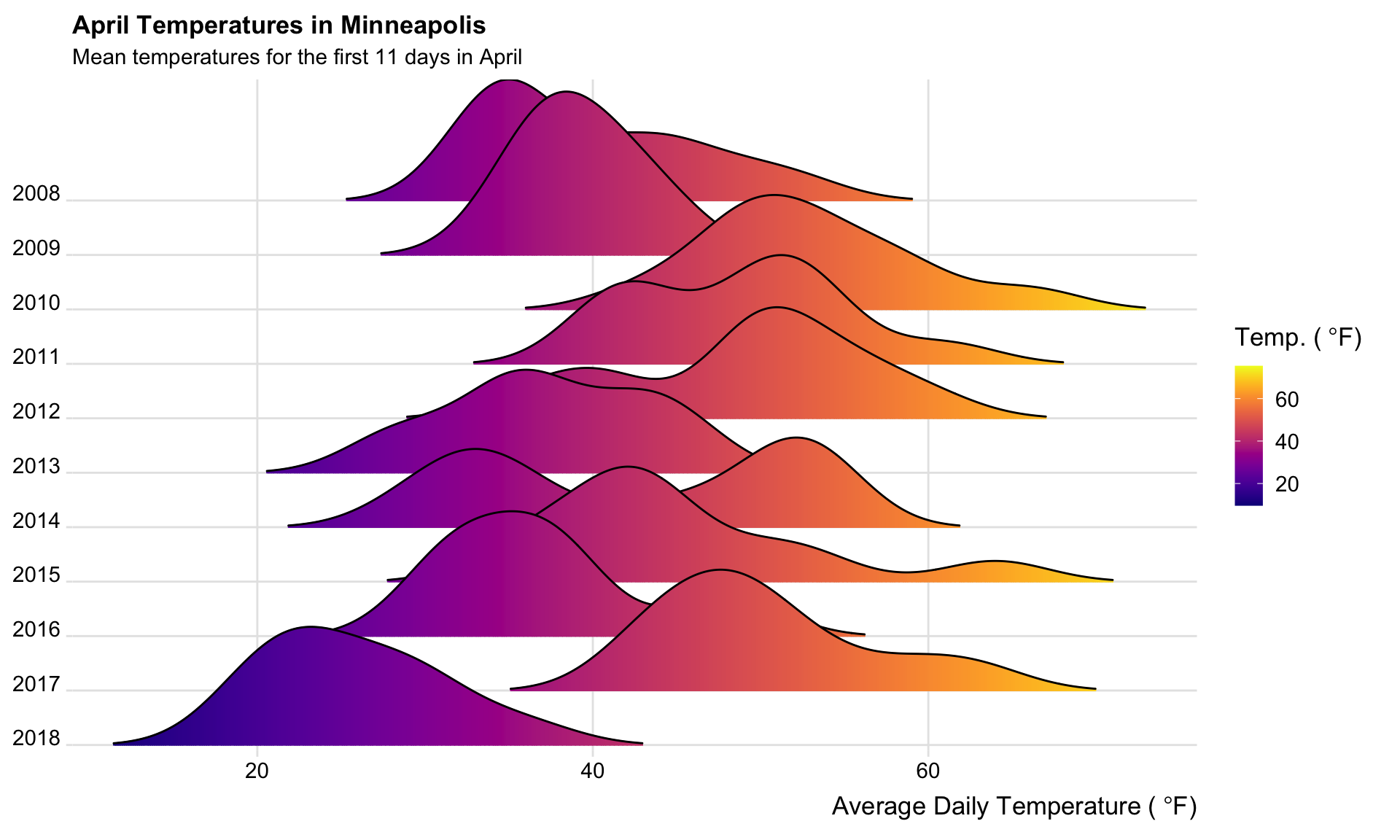# Spring Weather in Minneapolis

R
Author

Andy

Published

April 11, 2018

It feels like this spring has been especially terrible weather-wise. We have gotten a lot of snow and it has been cold. To evaluate whether this is the case or whether I have hindsight bias, I pulled some historical weather data for the month of April from Weather Underground.

``````library(dplyr)
library(forcats)
library(ggplot2)
library(ggridges)
library(viridis)

# Filter dates
april = april %>%
filter(date <= 11)``````

I grabbed data back to 2008 (available at https://raw.githubusercontent.com/zief0002/Public-Stuff/master/data/april-weather.csv). To be fair about the comparisons I was making, I filtered the data so that I only had the first eleven days of April for each year represented in the data. (April is a month in Minnesota that has a lot of variation from the first to last day of the month.) I then counted the number of those days each year that it snowed, as well as, the number of days over 40℉, 50℉, and 60℉.

``````april %>%
group_by(year) %>%
summarize(
days_with_snow = length(grep(pattern = "Snow", x = events)),
days_over_40 = sum(temp_high > 40),
days_over_50 = sum(temp_high > 50),
days_over_60 = sum(temp_high > 60)
)``````
``````# A tibble: 11 × 5
year days_with_snow days_over_40 days_over_50 days_over_60
<dbl>          <int>        <int>        <int>        <int>
1  2008              4            7            3            2
2  2009              3           10            4            0
3  2010              0           11           11            7
4  2011              2           11            9            4
5  2012              0           11            9            6
6  2013              4            7            2            0
7  2014              3            8            6            4
8  2015              2           11            6            3
9  2016              3            8            2            1
10  2017              2           11           11            4
11  2018              5            1            0            0``````

This suggested that my snow hypothesis (we have had more snow this year) may be erroneous. But, we have had a couple large snows; maybe we had more snow on the days we actually had snow. To evaluate this, I computed the total amount of precipitation for the years it actually snowed during the first eleven days of April.

``````# Get the case numbers for the days that "Snow" is in the event column
snow_days = grep(pattern = "Snow", x = april\$events)

# Compute the total amount of precipitation on the days with snow
april %>%
filter(row_number() %in% snow_days) %>%
group_by(year) %>%
summarize(
total_snow = sum(precip, na.rm = TRUE)
)``````
``````# A tibble: 9 × 2
year total_snow
<dbl>      <dbl>
1  2008       0.96
2  2009       0.47
3  2011       0.08
4  2013       1.39
5  2014       0.81
6  2015       0.71
7  2016       0.06
8  2017       0.18
9  2018       0.82``````

While more snow than the last couple years, 2018 doesn’t stand out as a banner April for the amount of snow. We will attribute this erroneous hypothesis to snow-blindness.

However, my “April = 🌡” hypothesis seems spot on. In 2018, so far, we have only had one day where the daily high temperatur was over 40℉. In the 10 years previous, almost all of the first eleven days of April hit over 40℉. This is compounded by the fact that the one day it went over 40℉, the temperature rose to a balmy 41℉!

Lastly, I looked at the distribution of the average daily temperatures since 2008. This was inspired by a post I saw here and here.

``````# Ridge plot of the distribution of average daily temperatures by year
ggplot(april, aes(x = temp_avg, y = fct_rev(factor(year)), fill = ..x..)) +
scale_x_continuous(expand = c(0.01, 0)) +
scale_y_discrete(expand = c(0.01, 0)) +
scale_fill_viridis(
name = expression(paste('Temp. (',~degree,'F)',sep='')),
option = "C"
) +
labs(
title = 'April Temperatures in Minneapolis',
subtitle = 'Mean temperatures for the first 11 days in April',
x = expression(paste('Average Daily Temperature (',~degree,'F)',sep=''))
) +
theme_ridges(
font_size = 13,
grid = TRUE
) +
theme(
axis.title.y = element_blank()
)``````
``````Warning: The dot-dot notation (`..x..`) was deprecated in ggplot2 3.4.0.